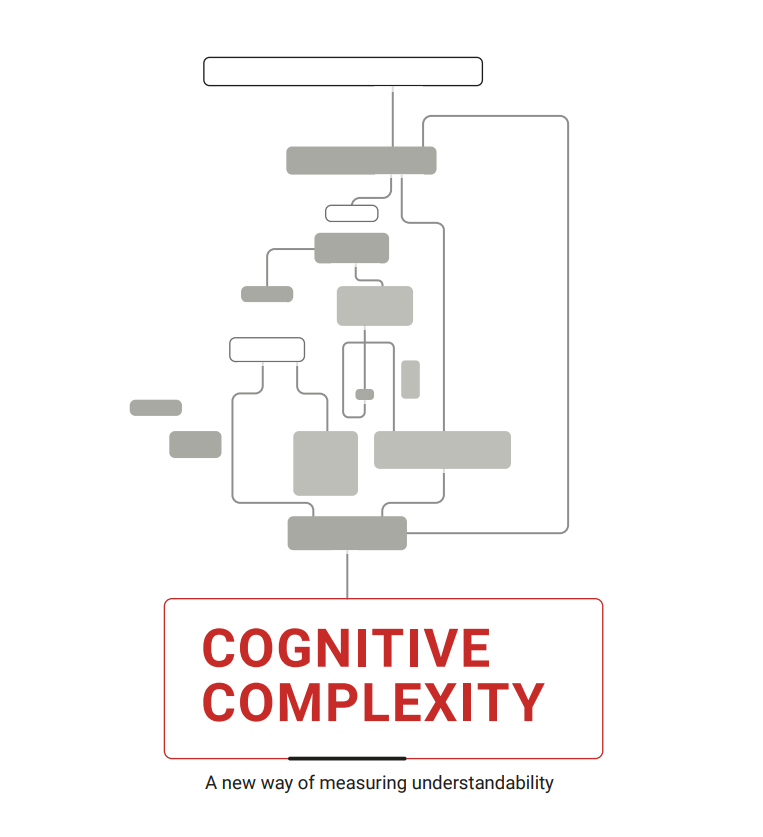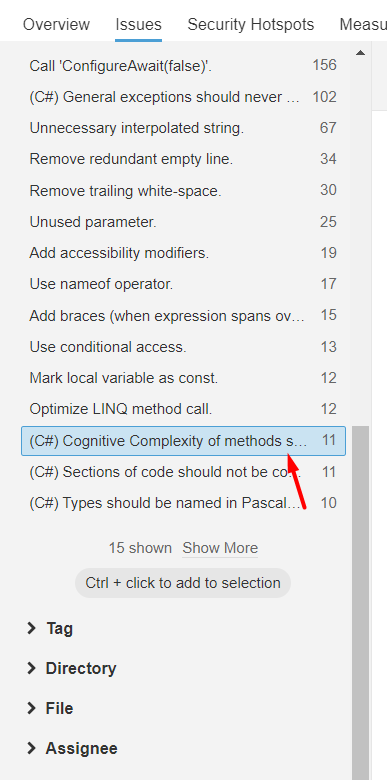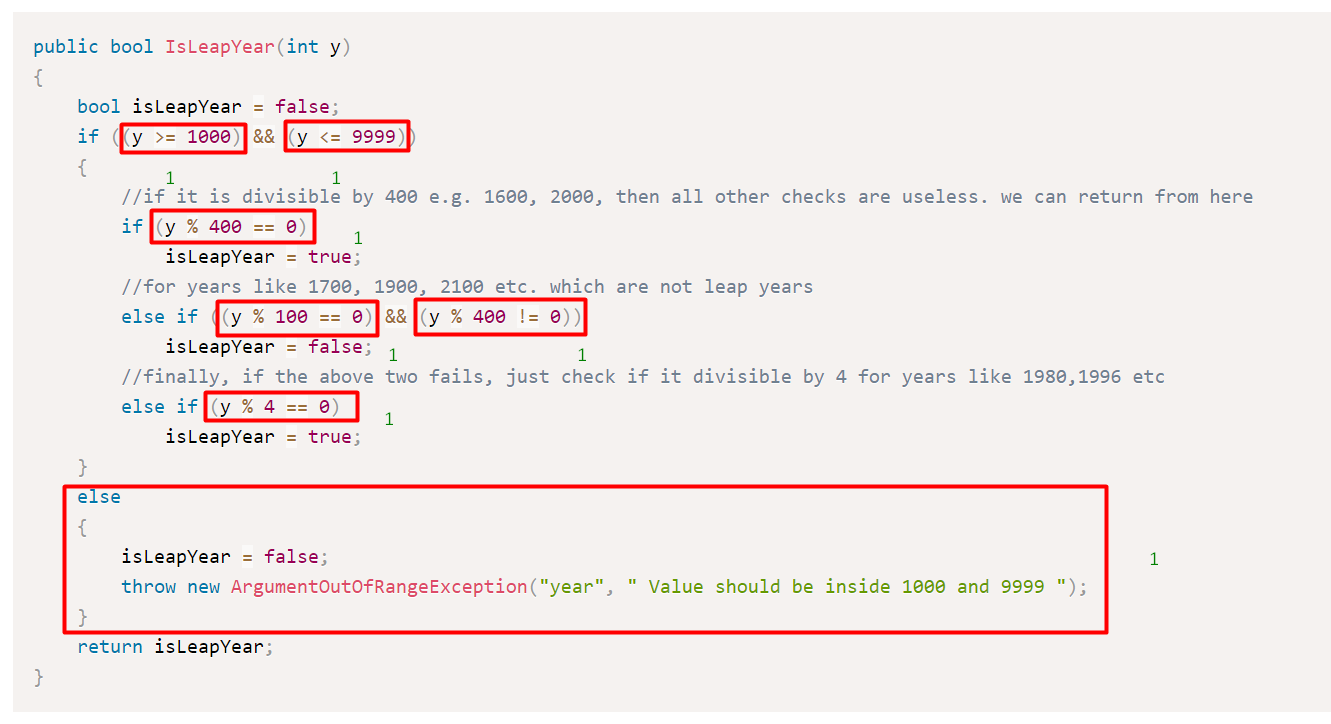# What is Cognitive Complexity in sonar report?Cognitive Complexity is a measure of how difficult a unit of code is to effortlessly understand . Unlike Cyclomatic Complexity, which determines how difficult your code will be to test, Cognitive Complexity tells you how difficult your code will be to read and understand.

Definition

``Cognitive Complexity, Because Testability != Understandability``

Your written code must be as simple one to understand as the above definition, simple.

``less Cognitive Complexity more Readability``

Where do we see Cognitive Complexity in SonarQube?

We can find cognitive complexity error in sonar as:

`Go to Project->Issues Tab->Rules Drop-down->Cognitive Complexity`How do we calculate Cognitive Complexity?

The first thing we should not need to do calculating by ourself but Cognitive Complexity can be done or maintained by SonarQube. Here we can see basic logic to calculate Cognitive Complexity.

Before knowing Cognitive Complexity we should know Cyclomatic Complexity. Let's take an example of checking leap year:

``public bool IsLeapYear(int y)  {      bool isLeapYear = false;      if ((y >= 1000) && (y <= 9999))      {          //if it is divisible by 400 e.g. 1600, 2000, then all other checks are useless. we can return from here          if (y % 400 == 0)              isLeapYear = true;          //for years like 1700, 1900, 2100 etc. which are not leap years          else if ((y % 100 == 0) && (y % 400 != 0))              isLeapYear = false;          //finally, if the above two fails, just check if it divisible by 4 for years like 1980,1996 etc          else if (y % 4 == 0)              isLeapYear = true;      }      else      {          isLeapYear = false;          throw new ArgumentOutOfRangeException("year", " Value should be inside 1000 and 9999 ");      }      return isLeapYear;  } ``

The Cyclomatic Complexity of the above function is 7. Basically, it tells us how many test cases needed to test this function and to have 100% code coverage:For Cognitive Complexity, the measuring algorithm would be slightly different and it will be some like following:

For each condition, it increases the count by 1 and if there is a break in the condition flow i.e. combination of && and ||, it further increases it. If there are 5 conditions all gated with AND, then the score will be 1, Although if there is combination of AND and OR, then for every break, the score will increase.